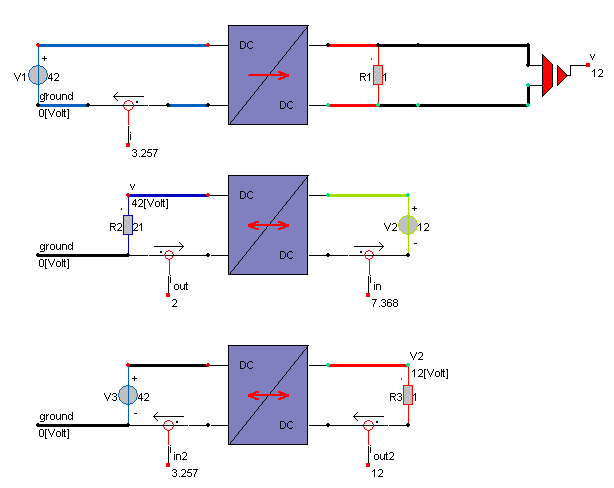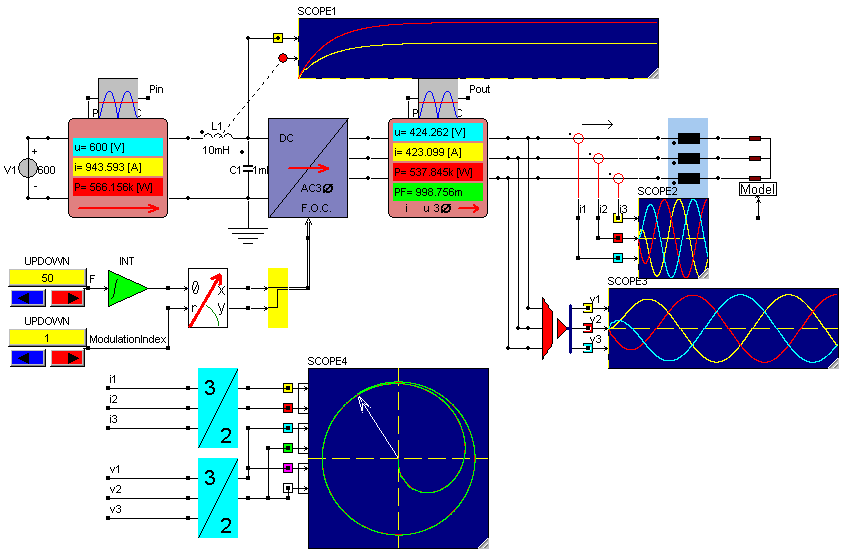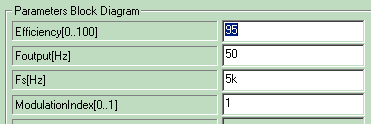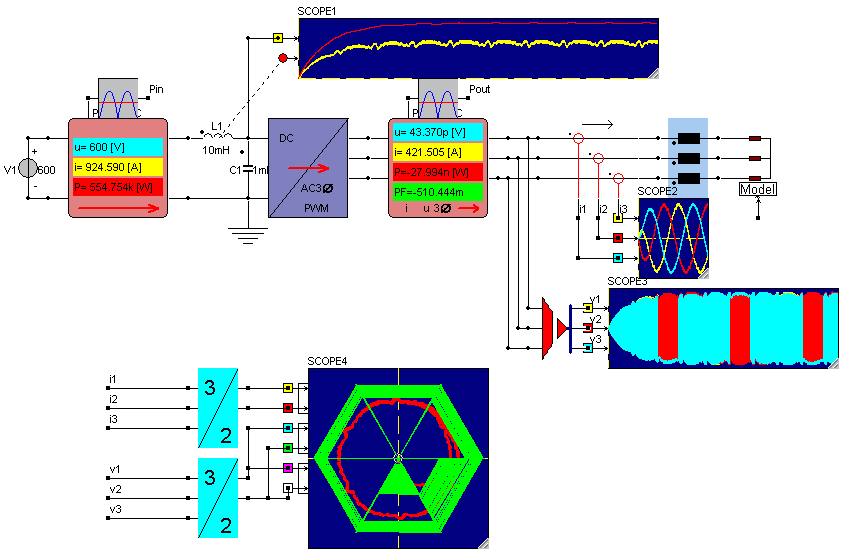• Introduction
• What is in this manual
• What is Caspoc
• User interface
• Introduction
• Starting
• Simulation
• Editing
• Viewing and printing
• Getting Started
• Basic editing
• Simulation in the time domain
• Basic User Interface Topics
• Editing
• Simulation
• Viewing
• Library
• Reports
• Project management
• Circuit and Block Diagram Components
• Introduction
• Cscript and user defined functions
• Component parameters
• Modeling Topics
• Introduction
• Power Electronics
• Semiconductors
• Electrical Machines
• Electrical drives
• Power Systems
• Mechanical Systems
• Thermal Systems
• Magnetic Circuits
• Green Energy
• Coupling to FEM
• Experimenter
• Analog hardware description language
• Embedded C code Export
• Coupling to Spice
• Small Signal Analysis
• Matlab coupling
• Tips and tricks
• Appendices

## Power converters, easy modeling and efficiency calculation.

To calculate the efficiency of a power system, DC or AC, power converter blocks are added, that model power electronics without going into detail. The purpose of these blocks is to set up a power conversion system in a fast way and to calculate the transient behavior of such a system and to evaluate the efficicency of the system. The blocks are in the library PowerConverters/SystemThe ideal DCDC converter is converting in only one direction, like the buck and boost converter. Also a bidirectional DCDC copnverter can be used as is shown by the second and third simulation in the above figure. Here the power flow is bidirectional.

The parameters for the converters are simply the input voltage, output voltage and the efficiency of the converter. Dynamic aspects can be added by adding DC bus capacitors and or filter inductors and capacitors. Since all blocks have electrical connections, all circuit components like V, I R, L C, etc, can directly be added to the blocks.

AC converters can directly be applied where the modulation type can be choosen. For example the Field Oriented Control approach is shown below.The required output voltage is directly controlled from the F.O.C. input which is a 2 phase vector input.

For PWM controlled inverters, only a basic set of parameters has to be defined, being the efficiency, output and switching frequency and the modulation index.The output from the PWM inverter are the true PWM modulated voltages.Sensors for measuring the power, voltage, current and power factor are in the library sensors.